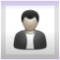# Latest news

1 of 1 message available2018-07-18 01:49:21 UTC (over 3 years ago)

I couldn't find a place to report this, so I thought I would try here.

Re: Figure 8.16. Example for layer mode “Overlay”

The explanation given is:

Overlay mode inverts the pixel value of the lower layer, multiplies it by two times the pixel value of the upper layer, adds that to the original pixel value of the lower layer, divides by 255, and then multiplies by the pixel value of the original lower layer and divides by 255 again. It darkens the image, but not as much as with “Multiply” mode.

As described above, the equation would be:

E = I / 255 x ( [ I + ( 2 x M ) x ( 255 – I ) ] / 255 )

The equation given by Help is:

E = I / 255 x [ I + ( 2 x M ) x ( 255 – I )/255 ]